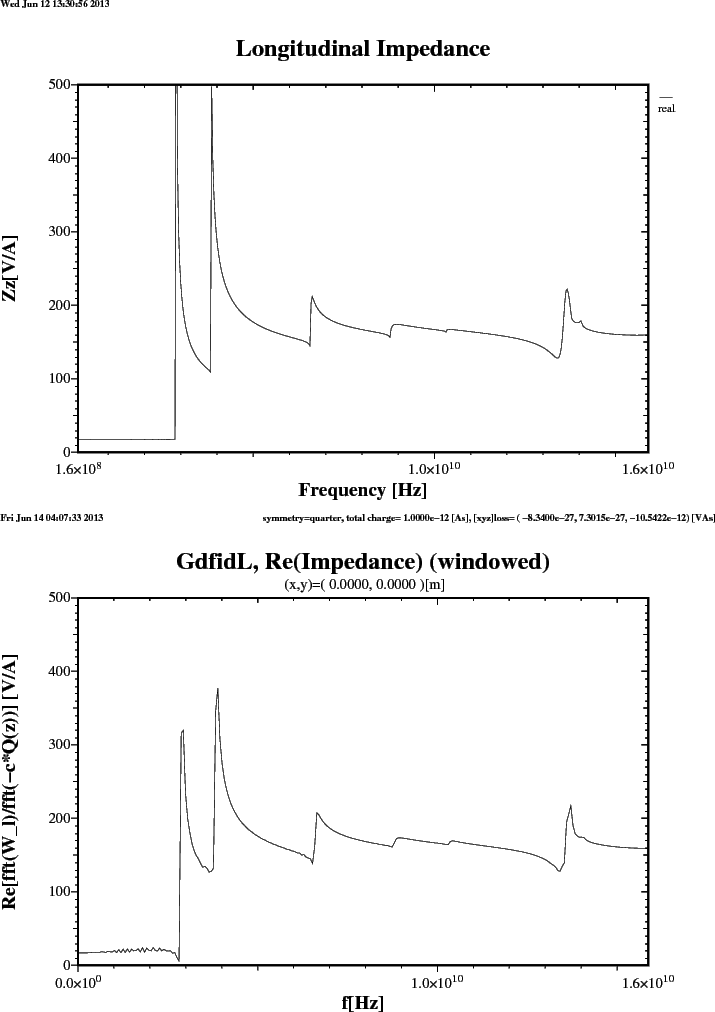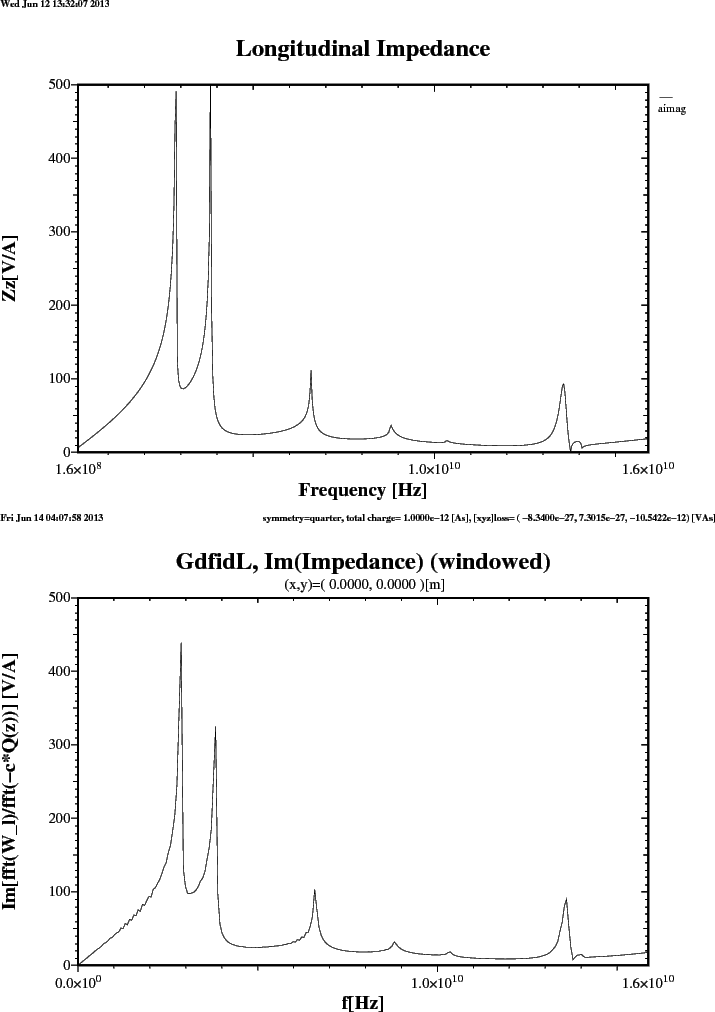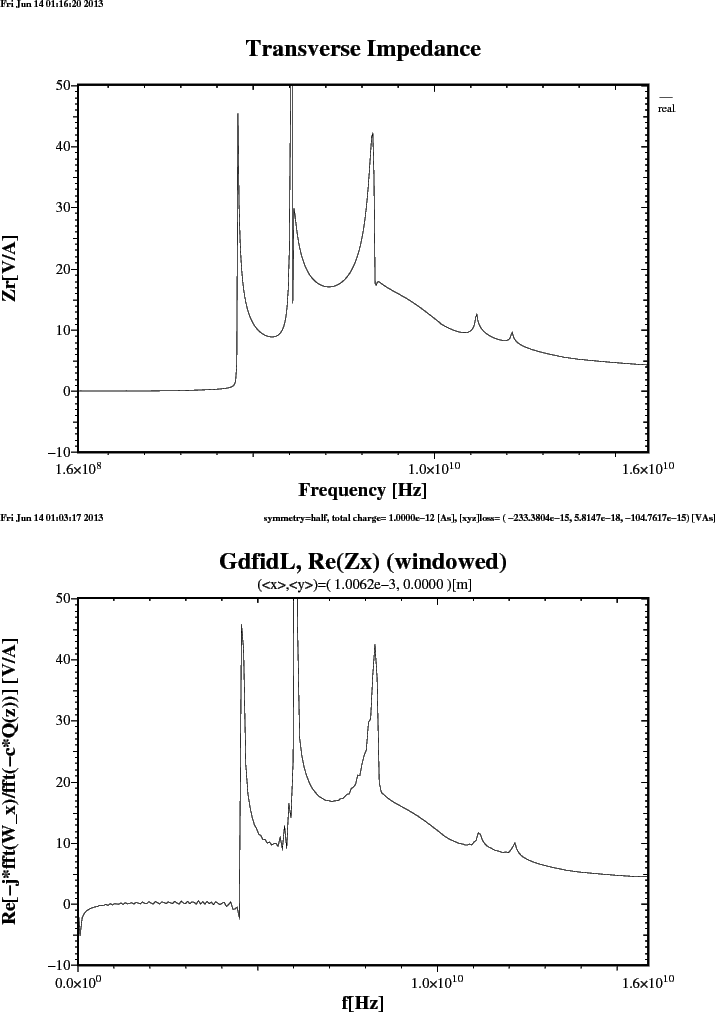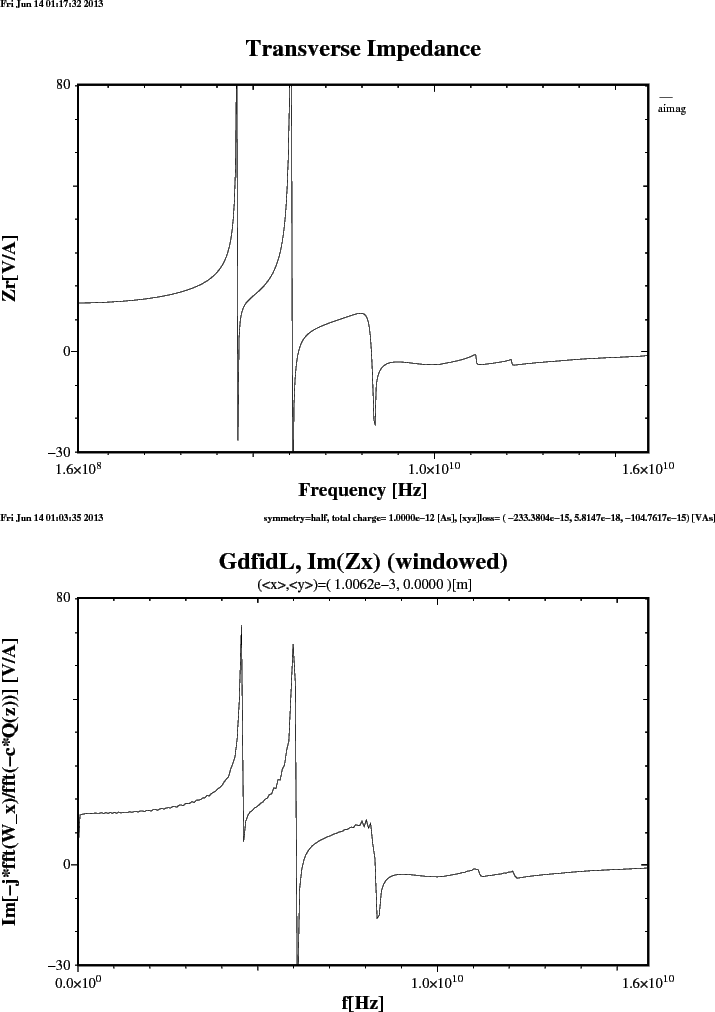# Kazi: Longitudinal and transverse Impedances of circular symmetric Structures.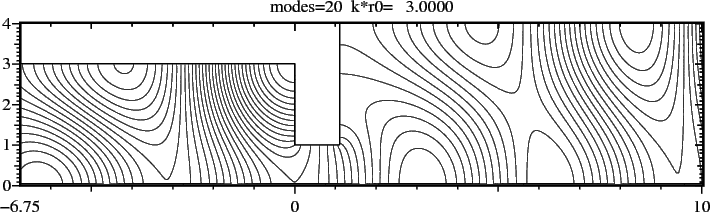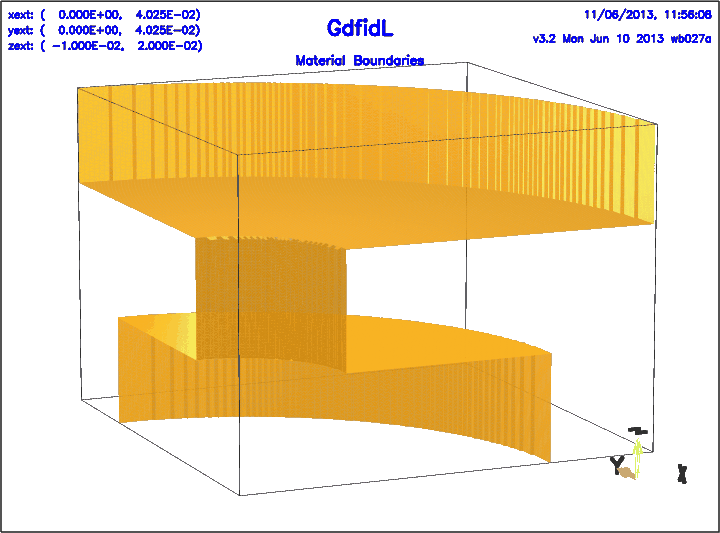This Writeup publishes the Program which came out of my Diploma Thesis. This was back in 1993. The Program is written in Fortran-77, with DOUBLE COMPLEX Extension. In Fortran-77, as then Fortran-90 Compilers were just emerging. The Program solves the resulting banded linear Systems using some self developed Out-Of-Core Solver, as back then, in 1993, 64 MBytes of RAM was what a High-End Workstation would have.

The Sourcecode for the Mode Matching Program Kazi. The Sourcecode for the Program which creates Fieldplots for m=0 Plot. The Sourcecode for the Program which re-formats the Results ReFormat.

A Shell-Script which generates some Inputfile etc and creates a Plot of Fieldlines is plot.x. The resulting Plot is shown in Figure 1.

A Shell-Script which generates some Inputfile, compiles the Program, and runs the Program for computing the longitudinal Impedance (m=0), is a.x. The Results are shown in Figures 3 .. 4. The Inputfile used to compute the longitudinal Impedance with GdfidL is 2Jumps-Wz.gdf.

A Shell-Script for computing the transverse Impedance (m=1), is ar.x. The Results are shown in Figures 5 .. 6.

The Inputfile used to compute the transverse Impedance with GdfidL is 2Jumps-Wx.gdf.